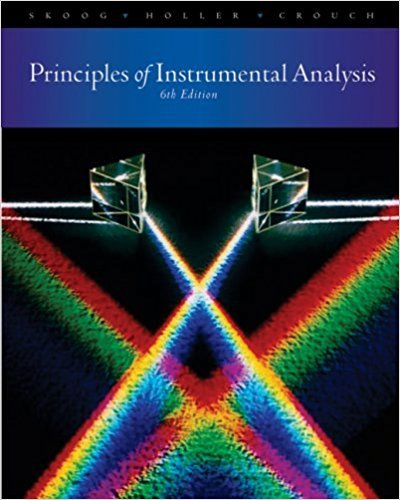×
×

# Define(a) elution(b) mobile phase(c) stationary phase(d) distribution constant(e)ISBN: 9780495012016 317

## Solution for problem 26-1 Chapter 26

Principles of Instrumental Analysis | 6th Edition

• Textbook Solutions
• 2901 Step-by-step solutions solved by professors and subject experts
• Get 24/7 help from StudySoup virtual teaching assistantsPrinciples of Instrumental Analysis | 6th Edition

4 5 0 335 Reviews
20
4
Problem 26-1

Define(a) elution(b) mobile phase(c) stationary phase(d) distribution constant(e) retention time(f) retention factor(g) selectivity factor(h) plate height(i) longitudinal diffusion(j) eddy diffusion(k) column resolution(I) eluent

Step-by-Step Solution:
Step 1 of 3

Chemistry (CHM 1045) Chapter One What is -A constantly changing science that impacts the Chemistry world on a daily basis -Ancient but by all means a modern science the study of matter and the changes it undergoes chemistry What is the Scientific Method a universal way...

Step 2 of 3

Step 3 of 3

##### ISBN: 9780495012016

The answer to “Define(a) elution(b) mobile phase(c) stationary phase(d) distribution constant(e) retention time(f) retention factor(g) selectivity factor(h) plate height(i) longitudinal diffusion(j) eddy diffusion(k) column resolution(I) eluent” is broken down into a number of easy to follow steps, and 23 words. Principles of Instrumental Analysis was written by and is associated to the ISBN: 9780495012016. This full solution covers the following key subjects: . This expansive textbook survival guide covers 34 chapters, and 619 solutions. This textbook survival guide was created for the textbook: Principles of Instrumental Analysis , edition: 6. Since the solution to 26-1 from 26 chapter was answered, more than 239 students have viewed the full step-by-step answer. The full step-by-step solution to problem: 26-1 from chapter: 26 was answered by , our top Chemistry solution expert on 03/02/18, 06:21PM.

Unlock Textbook Solution